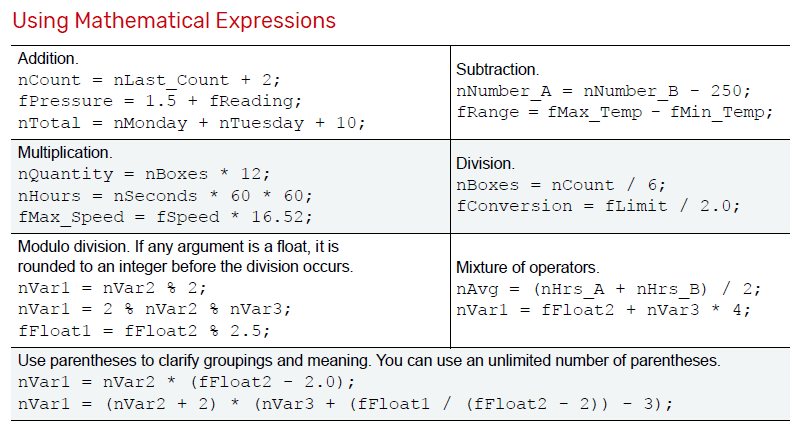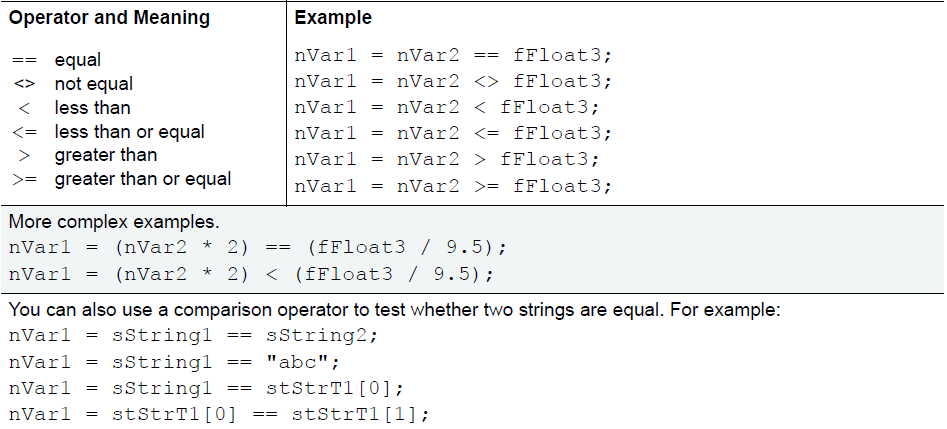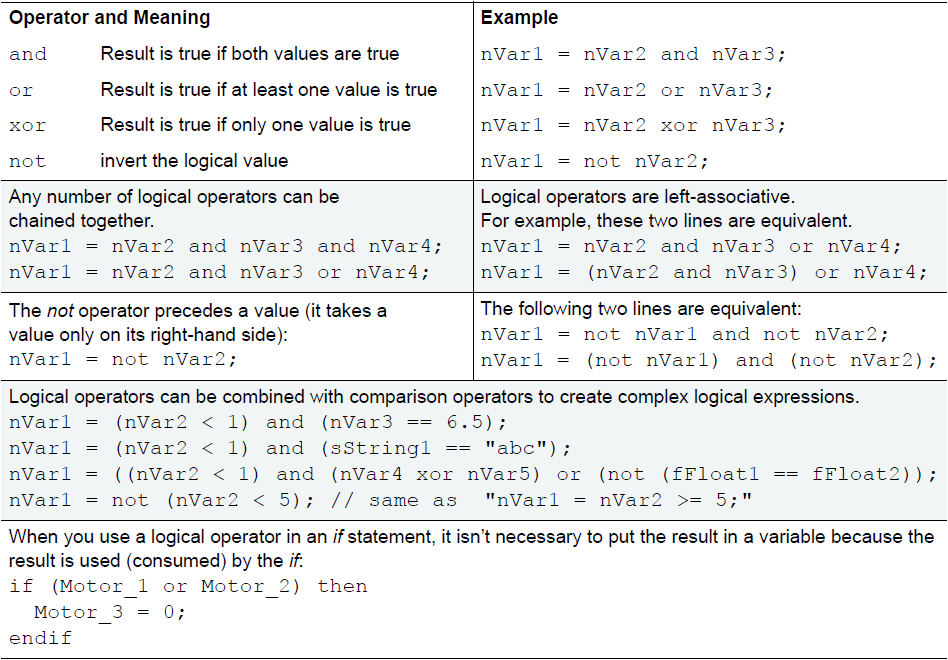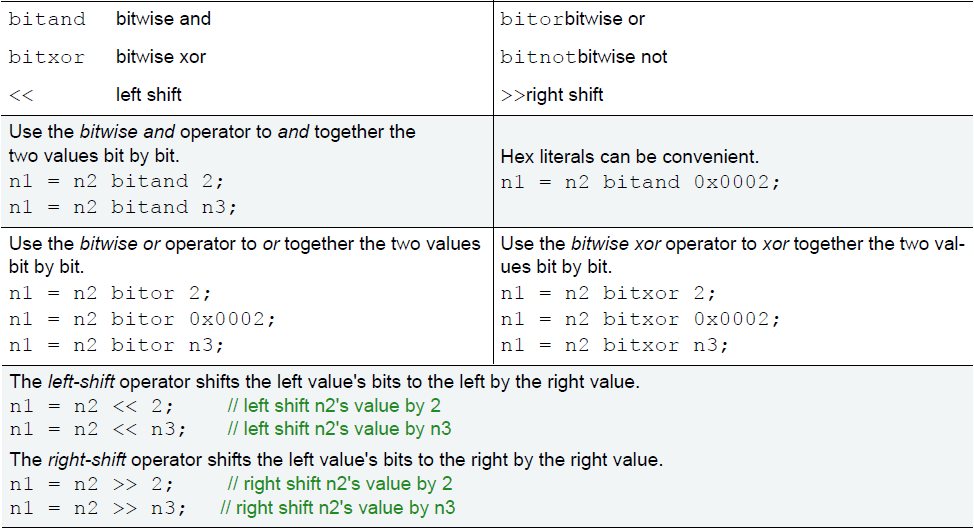## Expressions and Operators

OptoScript includes mathematical expressions as well as comparison, logical, and bitwise operators. Because expressions and operators are built into the OptoScript language, several PAC Control commands such as Multiply, Bit Shift, and Greater Than or Equal? aren’t used.

Here are some examples:### Using Comparison Operators

Comparison operators are used to compare two numbers. They will either return false (an Integer 32 value of zero) or true (an Integer 32 value of non-zero). OptoScript supports the following comparison operators for comparing two numeric values:

NOTE: Except for the equal operator (==), the comparison operators can be used only with a numeric expression. They can't be used with a null which isn't considered to be a number.### Using Logical Operators

All OptoScript logical operators return false (an Integer 32 value of zero) or true (an Integer 32 value of non-zero). OptoScript supports the following logical operators for numeric values:### Using Bitwise Operators

All OptoScript bitwise operators operate on integer values. OptoScript supports these bitwise operators:#### Summary

Learn how OptoScript uses mathematical expressions as well as comparison, logical, and bitwise operators.